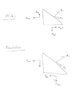# What is the proper form for Mohr's Circle (rotation of axes) calculation?

swampwiz
I'm getting conflicting information on the proper form of Mohr's Circle for the stress of a system at a rotation from nominal.

Actually it seems that Mohr's Circle is not a tool for just stress or moment if area/stress calculation, but in general for a 2-D simple symmetric form eigenproblem

[ K ]{ x } = λ{ x }

[ K ] → 2-D symmetric matrix

but this analysis seems to only be used in stress or moment if area/stress calculation, I will ask it here as a mechanical engineering topic rather than as a linear algebra topic.

Anyway, it seems that the rotation of axes for a static vector (i.e., the vector stays the same, only the coordinate system measuring it changes) has the form of

[ Ω ] =

[ C S ]
[ -S C ]

which is the transpose (and therefore inverse) of the rotation of a vector within a static coordinate system - and it is this minutiae of detail that I think its causing the problem.

So this rotation matrix becomes the eigenvector matrix for the previously mentioned eigenproblem, as a rotation from the principle aces representation (i.e., [ K ] as a diagonal matrix [ λ ] , which would be the eigenvalues.)

[ λ ] = [ Ω ]T [ K ] [ Ω ]

[ K ] = [ Ω ] [ λ ] [ Ω ]T

So from any given original [ K], the matrix for rotated axes [ K' ] would be

[ K' ] = [ Ω ] [ K ] [ Ω ]T

So going through the math, there is

let X = ½ ( kxx - kyy )

let Y = kxy

let I = cos( 2 θ ) X + sin( 2 θ ) Y

let J = - sin( 2 θ ) X + cos( 2 θ ) Y

λAVG = ½ ( kxx + kyy )

kxx' = λAVG + I

kyy' = λAVG - I

kyy' = J

Or at least that it what I come up with - and seems to correlate with that referenced @ foundationcoalition.org - http://www.foundationcoalition.org/resources/en/engr214/Chapter5.pdf (page 5 in the document, or 109 per the header.) However at Wikipedia at an article for moments of inertia - http://en.wikipedia.org/wiki/Second_moment_of_area#Axis_rotation_in_the_xy_plane - the rotation has the signs of the sin terms reversed, which would indicate that the rotation matrix should be inverted - even though that article has the proper rotation matrix!

So my question is which if these references is correct? The foundationcoalition.org document (which my analysis seems to be in agreement), or the Wikipedia article?

If the Wikipedia is, as I suspect incorrect, I will change it.

Studiot
Wiki is correct

look at equation 5.11 of your first (pdf) reference. There is a leading minus sign to the first term that I think should not be there.

swampwiz
Wiki is correct

look at equation 5.11 of your first (pdf) reference. There is a leading minus sign to the first term that I think should not be there.

If that is the case, then sin term in EQ 5.10 should be negative as well.

I've researched at least 2 books that supports my contention (Foundations of Solid Mechanics, Fung; Intermediate Mechanics of Materials, Barber), so I am going to consider my contention correct and will change the Wikipedia article.

Last edited:
Studiot
If that is the case, then sin term in EQ 5.10 should be negative as well

Yes that is correct the sin term is negative in equation 5.10.

But if you look carefully at the directions of the contributions Wiki and Foundation are referring to different situations.

I have sketched the stress systems comparable for each, rather than try to compare a moment of inertia with a stress. Note that the shear directions are reversed.

#### Attachments

•stress_system1.jpg
7.4 KB · Views: 412
swampwiz
OK, so it appears that this a coordinate system issue. I've always thought that the rotation of axes should be as I've described.